Elementary Algebra

# Review Exercises

Elementary AlgebraReview Exercises

### Review Exercises

##### Rectangular Coordinate System

Plot Points in a Rectangular Coordinate System

In the following exercises, plot each point in a rectangular coordinate system.

556.

$(−1,−5)(−1,−5)$
$(−3,4)(−3,4)$
$(2,−3)(2,−3)$
$(1,52)(1,52)$

557.

$(4,3)(4,3)$
$(−4,3)(−4,3)$
$(−4,−3)(−4,−3)$
$(4,−3)(4,−3)$

558.

$(−2,0)(−2,0)$
$(0,−4)(0,−4)$
$(0,5)(0,5)$
$(3,0)(3,0)$

559.

$(2,32)(2,32)$
$(3,43)(3,43)$
$(13,−4)(13,−4)$
$(12,−5)(12,−5)$

Identify Points on a Graph

In the following exercises, name the ordered pair of each point shown in the rectangular coordinate system.

560.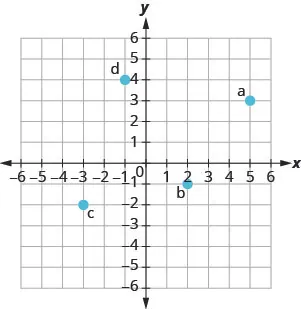561.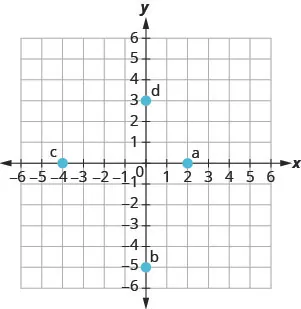Verify Solutions to an Equation in Two Variables

In the following exercises, which ordered pairs are solutions to the given equations?

562.

$5 x + y = 10 5 x + y = 10$

$(5,1)(5,1)$
$(2,0)(2,0)$
$(4,−10)(4,−10)$

563.

$y = 6 x − 2 y = 6 x − 2$

$(1,4)(1,4)$
$(13,0)(13,0)$
$(6,−2)(6,−2)$

Complete a Table of Solutions to a Linear Equation in Two Variables

In the following exercises, complete the table to find solutions to each linear equation.

564.

$y = 4 x − 1 y = 4 x − 1$

 $xx$ $yy$ $(x,y)(x,y)$ 0 1 $−2−2$
565.

$y = − 1 2 x + 3 y = − 1 2 x + 3$

 $xx$ $yy$ $(x,y)(x,y)$ 0 4 $−2−2$
566.

$x + 2 y = 5 x + 2 y = 5$

 $xx$ $yy$ $(x,y)(x,y)$ 0 1 $−1−1$
567.

$3 x + 2 y = 6 3 x + 2 y = 6$

 $xx$ $yy$ $(x,y)(x,y)$ 0 0 $−2−2$

Find Solutions to a Linear Equation in Two Variables

In the following exercises, find three solutions to each linear equation.

568.

$x + y = 3 x + y = 3$

569.

$x + y = −4 x + y = −4$

570.

$y = 3 x + 1 y = 3 x + 1$

571.

$y = − x − 1 y = − x − 1$

##### Graphing Linear Equations

Recognize the Relation Between the Solutions of an Equation and its Graph

In the following exercises, for each ordered pair, decide:

1. Is the ordered pair a solution to the equation?
2. Is the point on the line?
572.

$y = − x + 4 y = − x + 4$

$(0,4)(0,4)$ $(−1,3)(−1,3)$

$(2,2)(2,2)$ $(−2,6)(−2,6)$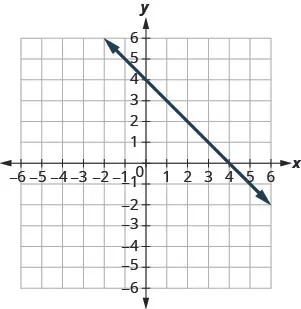573.

$y = 2 3 x − 1 y = 2 3 x − 1$

$(0,−1)(0,−1)$ (3, 1)

$(−3,−3)(−3,−3)$ (6, 4)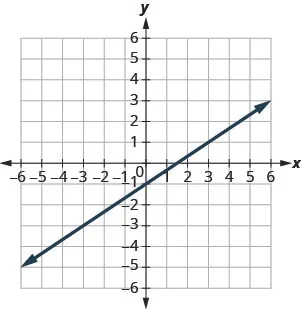Graph a Linear Equation by Plotting Points

In the following exercises, graph by plotting points.

574.

$y = 4 x − 3 y = 4 x − 3$

575.

$y = −3 x y = −3 x$

576.

$y = 1 2 x + 3 y = 1 2 x + 3$

577.

$x − y = 6 x − y = 6$

578.

$2 x + y = 7 2 x + y = 7$

579.

$3 x − 2 y = 6 3 x − 2 y = 6$

Graph Vertical and Horizontal lines

In the following exercises, graph each equation.

580.

$y = −2 y = −2$

581.

$x = 3 x = 3$

In the following exercises, graph each pair of equations in the same rectangular coordinate system.

582.

$y=−2xy=−2x$ and $y=−2y=−2$

583.

$y=43xy=43x$ and $y=43y=43$

##### Graphing with Intercepts

Identify the x- and y-Intercepts on a Graph

In the following exercises, find the x- and y-intercepts.

584.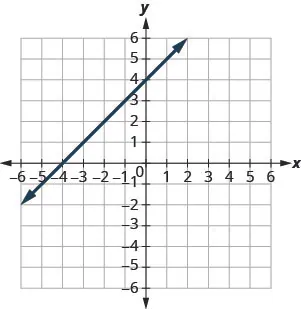585.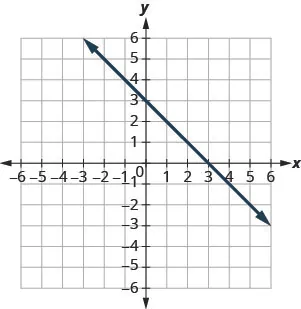Find the x- and y-Intercepts from an Equation of a Line

In the following exercises, find the intercepts of each equation.

586.

$x + y = 5 x + y = 5$

587.

$x − y = −1 x − y = −1$

588.

$x + 2 y = 6 x + 2 y = 6$

589.

$2 x + 3 y = 12 2 x + 3 y = 12$

590.

$y = 3 4 x − 12 y = 3 4 x − 12$

591.

$y = 3 x y = 3 x$

Graph a Line Using the Intercepts

In the following exercises, graph using the intercepts.

592.

$− x + 3 y = 3 − x + 3 y = 3$

593.

$x + y = −2 x + y = −2$

594.

$x − y = 4 x − y = 4$

595.

$2 x − y = 5 2 x − y = 5$

596.

$2 x − 4 y = 8 2 x − 4 y = 8$

597.

$y = 2 x y = 2 x$

##### Slope of a Line

Use Geoboards to Model Slope

In the following exercises, find the slope modeled on each geoboard.

598.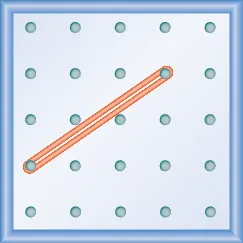599.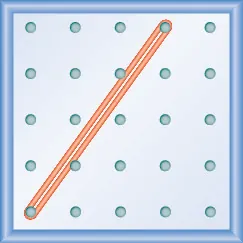600.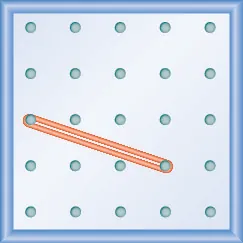601.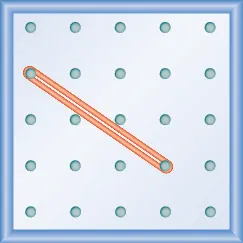In the following exercises, model each slope. Draw a picture to show your results.

602.

$1 3 1 3$

603.

$3 2 3 2$

604.

$− 2 3 − 2 3$

605.

$− 1 2 − 1 2$

Use $m=riserunm=riserun$ to find the Slope of a Line from its Graph

In the following exercises, find the slope of each line shown.

606.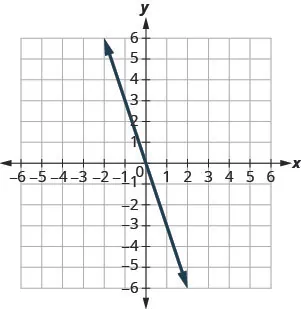607.608.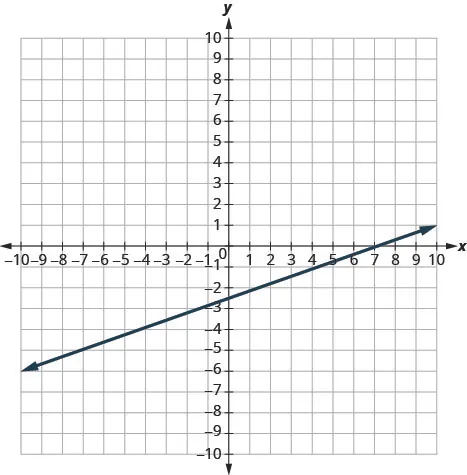609.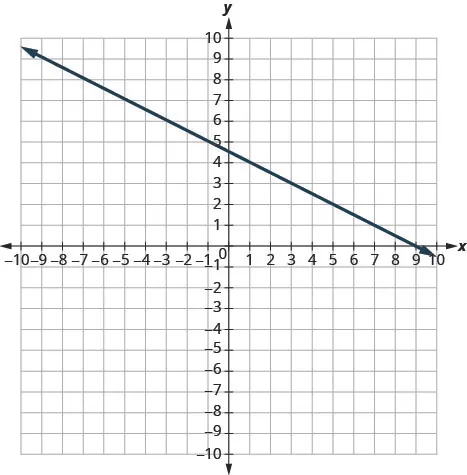Find the Slope of Horizontal and Vertical Lines

In the following exercises, find the slope of each line.

610.

$y = 2 y = 2$

611.

$x = 5 x = 5$

612.

$x = −3 x = −3$

613.

$y = −1 y = −1$

Use the Slope Formula to find the Slope of a Line between Two Points

In the following exercises, use the slope formula to find the slope of the line between each pair of points.

614.

$( −1 , −1 ) , ( 0 , 5 ) ( −1 , −1 ) , ( 0 , 5 )$

615.

$( 3 , 5 ) , ( 4 , −1 ) ( 3 , 5 ) , ( 4 , −1 )$

616.

$( −5 , −2 ) , ( 3 , 2 ) ( −5 , −2 ) , ( 3 , 2 )$

617.

$( 2 , 1 ) , ( 4 , 6 ) ( 2 , 1 ) , ( 4 , 6 )$

Graph a Line Given a Point and the Slope

In the following exercises, graph each line with the given point and slope.

618.

$(2,−2)(2,−2)$; $m=52m=52$

619.

$(−3,4)(−3,4)$; $m=−13m=−13$

620.

x-intercept $−4−4$; $m=3m=3$

621.

y-intercept 1; $m=−34m=−34$

Solve Slope Applications

In the following exercises, solve these slope applications.

622.

The roof pictured below has a rise of 10 feet and a run of 15 feet. What is its slope?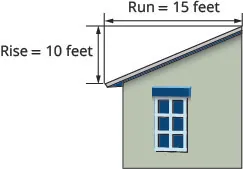623.

A mountain road rises 50 feet for a 500-foot run. What is its slope?

##### Intercept Form of an Equation of a Line

Recognize the Relation Between the Graph and the Slope–Intercept Form of an Equation of a Line

In the following exercises, use the graph to find the slope and y-intercept of each line. Compare the values to the equation $y=mx+by=mx+b$.

624.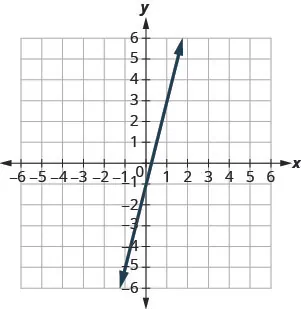$y = 4 x − 1 y = 4 x − 1$

625.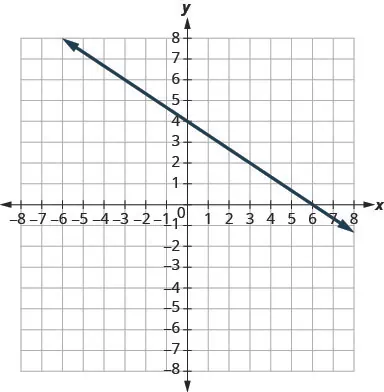$y = − 2 3 x + 4 y = − 2 3 x + 4$

Identify the Slope and y-Intercept from an Equation of a Line

In the following exercises, identify the slope and y-intercept of each line.

626.

$y = −4 x + 9 y = −4 x + 9$

627.

$y = 5 3 x − 6 y = 5 3 x − 6$

628.

$5 x + y = 10 5 x + y = 10$

629.

$4 x − 5 y = 8 4 x − 5 y = 8$

Graph a Line Using Its Slope and Intercept

In the following exercises, graph the line of each equation using its slope and y-intercept.

630.

$y = 2 x + 3 y = 2 x + 3$

631.

$y = − x − 1 y = − x − 1$

632.

$y = − 2 5 x + 3 y = − 2 5 x + 3$

633.

$4 x − 3 y = 12 4 x − 3 y = 12$

In the following exercises, determine the most convenient method to graph each line.

634.

$x = 5 x = 5$

635.

$y = −3 y = −3$

636.

$2 x + y = 5 2 x + y = 5$

637.

$x − y = 2 x − y = 2$

638.

$y = x + 2 y = x + 2$

639.

$y = 3 4 x − 1 y = 3 4 x − 1$

Graph and Interpret Applications of Slope–Intercept

640.

Katherine is a private chef. The equation $C=6.5m+42C=6.5m+42$ models the relation between her weekly cost, C, in dollars and the number of meals, m, that she serves.

1. Find Katherine’s cost for a week when she serves no meals.
2. Find the cost for a week when she serves 14 meals.
3. Interpret the slope and C-intercept of the equation.
4. Graph the equation.
641.

Marjorie teaches piano. The equation $P=35h−250P=35h−250$ models the relation between her weekly profit, P, in dollars and the number of student lessons, s, that she teaches.

1. Find Marjorie’s profit for a week when she teaches no student lessons.
2. Find the profit for a week when she teaches 20 student lessons.
3. Interpret the slope and P–intercept of the equation.
4. Graph the equation.

Use Slopes to Identify Parallel Lines

In the following exercises, use slopes and y-intercepts to determine if the lines are parallel.

642.

$4 x − 3 y = −1 ; y = 4 3 x − 3 4 x − 3 y = −1 ; y = 4 3 x − 3$

643.

$2 x − y = 8 ; x − 2 y = 4 2 x − y = 8 ; x − 2 y = 4$

Use Slopes to Identify Perpendicular Lines

In the following exercises, use slopes and y-intercepts to determine if the lines are perpendicular.

644.

$y = 5 x − 1 ; 10 x + 2 y = 0 y = 5 x − 1 ; 10 x + 2 y = 0$

645.

$3 x − 2 y = 5 ; 2 x + 3 y = 6 3 x − 2 y = 5 ; 2 x + 3 y = 6$

##### Find the Equation of a Line

Find an Equation of the Line Given the Slope and y-Intercept

In the following exercises, find the equation of a line with given slope and y-intercept. Write the equation in slope–intercept form.

646.

slope $1313$ and $y-intercepty-intercept$ $(0,−6)(0,−6)$

647.

slope $−5−5$ and $y-intercepty-intercept$ $(0,−3)(0,−3)$

648.

slope $00$ and $y-intercepty-intercept$ $(0,4)(0,4)$

649.

slope $−2−2$ and $y-intercepty-intercept$ $(0,0)(0,0)$

In the following exercises, find the equation of the line shown in each graph. Write the equation in slope–intercept form.

650.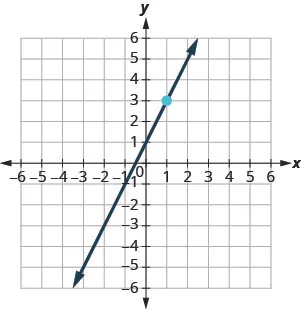651.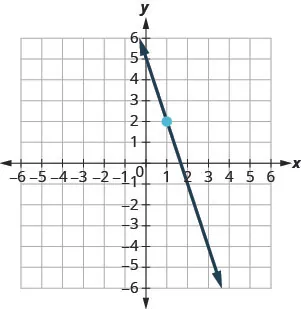652.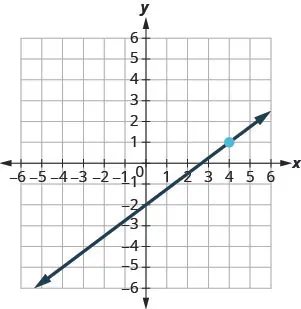653.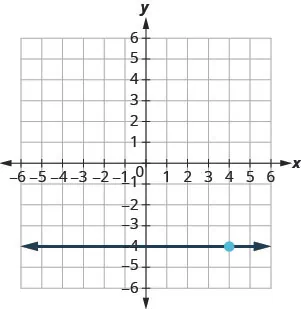Find an Equation of the Line Given the Slope and a Point

In the following exercises, find the equation of a line with given slope and containing the given point. Write the equation in slope–intercept form.

654.

$m=−14m=−14$, point $(−8,3)(−8,3)$

655.

$m=35m=35$, point $(10,6)(10,6)$

656.

Horizontal line containing $(−2,7)(−2,7)$

657.

$m=−2m=−2$, point $(−1,−3)(−1,−3)$

Find an Equation of the Line Given Two Points

In the following exercises, find the equation of a line containing the given points. Write the equation in slope–intercept form.

658.

$(2,10)(2,10)$ and $(−2,−2)(−2,−2)$

659.

$(7,1)(7,1)$ and $(5,0)(5,0)$

660.

$(3,8)(3,8)$ and $(3,−4)(3,−4)$.

661.

$(5,2)(5,2)$ and $(−1,2)(−1,2)$

Find an Equation of a Line Parallel to a Given Line

In the following exercises, find an equation of a line parallel to the given line and contains the given point. Write the equation in slope–intercept form.

662.

line $y=−3x+6y=−3x+6$, point $(1,−5)(1,−5)$

663.

line $2x+5y=−102x+5y=−10$, point $(10,4)(10,4)$

664.

line $x=4x=4$, point $(−2,−1)(−2,−1)$

665.

line $y=−5y=−5$, point $(−4,3)(−4,3)$

Find an Equation of a Line Perpendicular to a Given Line

In the following exercises, find an equation of a line perpendicular to the given line and contains the given point. Write the equation in slope–intercept form.

666.

line $y=−45x+2y=−45x+2$, point $(8,9)(8,9)$

667.

line $2x−3y=92x−3y=9$, point $(−4,0)(−4,0)$

668.

line $y=3y=3$, point $(−1,−3)(−1,−3)$

669.

line $x=−5x=−5$ point $(2,1)(2,1)$

##### Graph Linear Inequalities

Verify Solutions to an Inequality in Two Variables

In the following exercises, determine whether each ordered pair is a solution to the given inequality.

670.

Determine whether each ordered pair is a solution to the inequality $y:

$(0,1)(0,1)$
$(−2,−4)(−2,−4)$
$(5,2)(5,2)$
$(3,−1)(3,−1)$
$(−1,−5)(−1,−5)$

671.

Determine whether each ordered pair is a solution to the inequality $x+y>4x+y>4$:

$(6,1)(6,1)$
$(−3,6)(−3,6)$
$(3,2)(3,2)$
$(−5,10)(−5,10)$
$(0,0)(0,0)$

Recognize the Relation Between the Solutions of an Inequality and its Graph

In the following exercises, write the inequality shown by the shaded region.

672.

Write the inequality shown by the graph with the boundary line $y=−x+2y=−x+2$.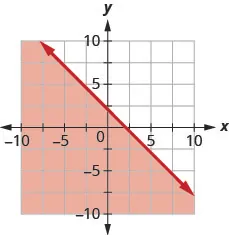673.

Write the inequality shown by the graph with the boundary line $y=23x−3y=23x−3$.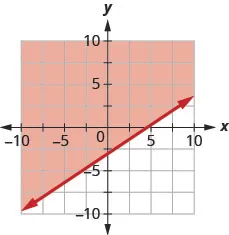674.

Write the inequality shown by the shaded region in the graph with the boundary line $x+y=−4x+y=−4$.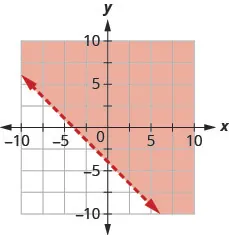675.

Write the inequality shown by the shaded region in the graph with the boundary line $x−2y=6.x−2y=6.$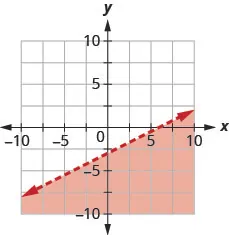Graph Linear Inequalities

In the following exercises, graph each linear inequality.

676.

Graph the linear inequality $y>25x−4y>25x−4$.

677.

Graph the linear inequality $y≤−14x+3y≤−14x+3$.

678.

Graph the linear inequality $x−y≤5x−y≤5$.

679.

Graph the linear inequality $3x+2y>103x+2y>10$.

680.

Graph the linear inequality $y≤−3xy≤−3x$.

681.

Graph the linear inequality $y<6y<6$.

Order a print copy

As an Amazon Associate we earn from qualifying purchases.